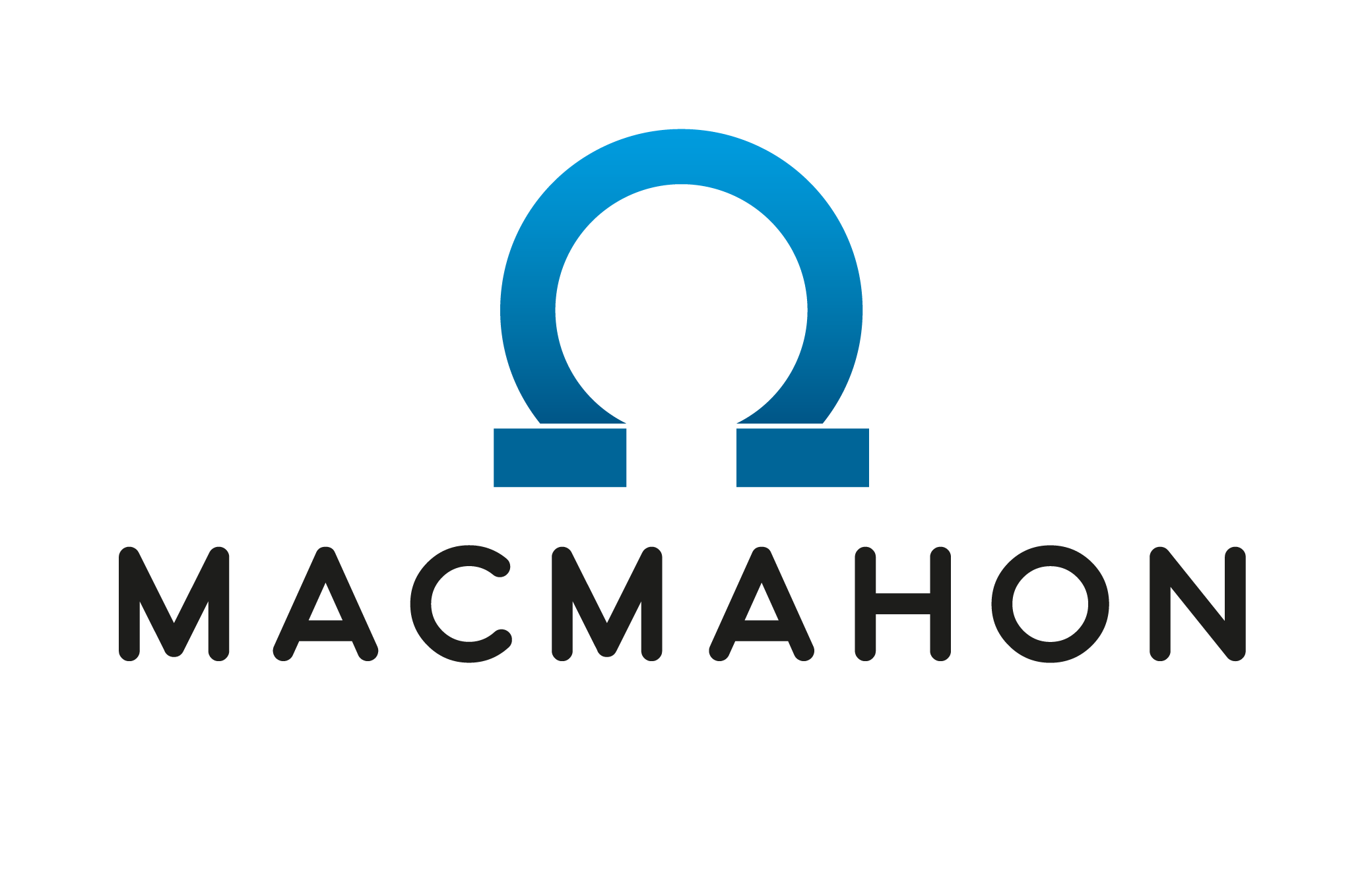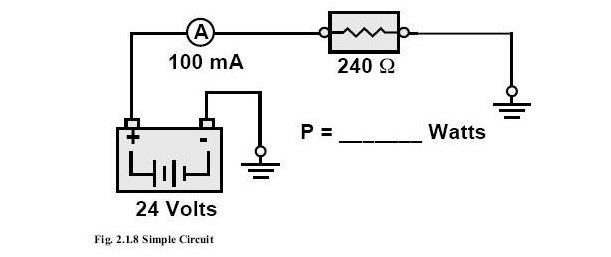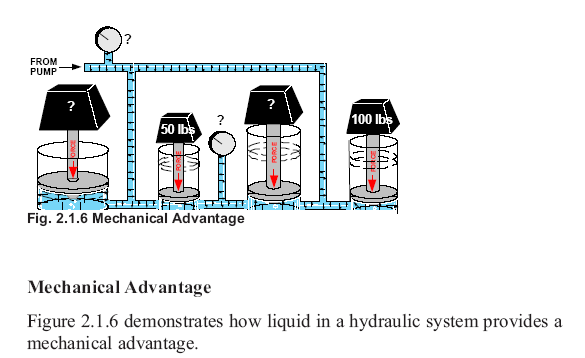### Question Title

#### * 13. Three helical gears running in line, in mesh. Gear A has 25 teeth and rotates @100rpm in a clockwise direction. Gear B has 75 teeth and gear C has 50 teeth.

 Clockwise Anti-Clockwise Sinusoidal 33.33 rpm 50 rpm 75 rpm 125 rpm A - What direction is gear C rotating? A - What direction is gear C rotating? Clockwise A - What direction is gear C rotating? Anti-Clockwise A - What direction is gear C rotating? Sinusoidal A - What direction is gear C rotating? 33.33 rpm A - What direction is gear C rotating? 50 rpm A - What direction is gear C rotating? 75 rpm A - What direction is gear C rotating? 125 rpm B - What is the speed of gear C? B - What is the speed of gear C? Clockwise B - What is the speed of gear C? Anti-Clockwise B - What is the speed of gear C? Sinusoidal B - What is the speed of gear C? 33.33 rpm B - What is the speed of gear C? 50 rpm B - What is the speed of gear C? 75 rpm B - What is the speed of gear C? 125 rpm C - What is the speed of gear B? C - What is the speed of gear B? Clockwise C - What is the speed of gear B? Anti-Clockwise C - What is the speed of gear B? Sinusoidal C - What is the speed of gear B? 33.33 rpm C - What is the speed of gear B? 50 rpm C - What is the speed of gear B? 75 rpm C - What is the speed of gear B? 125 rpm

### Question Title

#### Calculating power in an electrical circuit### Question Title

#### Question TitleT